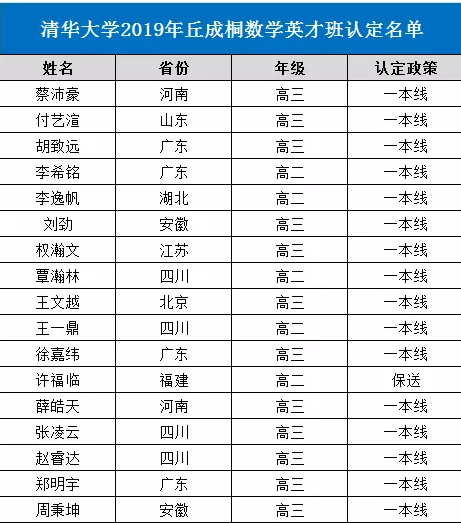最新的消息!清华大学2020年丘成桐数学英才班招生计划将于10月份启动，想要报名参加的同学赶紧来看看相关的详情。大家都知道，清华大学的丘成桐数学英才班招收的是在数学方面具有一定的特长的学生，面向的年级是高中二年级和高中三年级，而且能够被录取的话，对于考生的自主招生帮助是比较大的。下面是2020年清华大学丘成桐数学英才班的招生信息，浙江秦学教育小编也给大家附上了2019年的真题，看看大家会不会做。一、2020清华丘成桐数学英才班招生即将启动

清华大学丘成桐数学科学中心发布消息，2020年“丘成桐数学英才班”招生工作即将于10月启动，欢迎学习成绩优异且在数学方面有超长表现的中学生朋友关注。

英才班招收对象为具有数学潜质和特长，并有志于从事数学研究的普通高中二年级在读学生及三年级应届毕业生。

请关注清华大学本科招生网(https://www.tsinghua.edu.cn/publish/bzw/)。报名系统启动后，选择“丘成桐数学英才班”进行报名。

英才班招生选拔程序包括初审、测试、认定、确认。

通过初审的学生，参加我校组织的测试。测试包括综合测试、学科能力测试(包括数学一试和数学二试)、心理测试、面试。

数学一试测试范围包括中学数学全部内容、微积分及线性代数，主要考察学生对数学基础知识的理解和掌握;

数学二试为非固定形式的开放性内容，现学现考，主要考察学生的学习和理解能力。

通过学科能力测试的学生进入面试环节，面试主要考察学生数学文化与知识面、数学思维能力及语言表达能力等综合素质。

测试结果“优秀”者将获得“丘成桐数学英才班”入围认定。英才班每年招收不超过30人。

二、2019年考试情况

2019清华英才班的复试分为两天，第一天是常规地考综合测试和心理测试，综合测试考语数外理化五科，心理测试一般是为了建立考生的性格模型。

据考生反馈，数学部分测试主要考察了数论、组合、立体几何等知识，一共7到大题，没有选择;心理测试则是90道选择，测试结果不计入总分。

第二天的测试是目前非常流行的一种测试方式——即现学现考，上午教授讲课，下午即进行测试。目前该种模式在2018年清华英才班复试时已经实行。

三、2019年考试真题(部分)

1、x(x+2)(x+4)=2^y * 3^z，求正整数解

2、n为偶数，n阶全图G所有边染成红蓝两色，求同色三角形数量最小值

3、.A为{1,2,……,N}子集

E(A)={(a,b,c,d)|a,b,c,d属于A，且a+b-c-d=0(modN)}

F(A)={(a,b,c,d)|a,b,c,d属于A，且a+b-c-d=2(modN)}

求证|E(A)|不小于|F(A)|，并指出取等

4、A1……An为n个有限集

求证 sigma|Ai∩Aj∩Ak|不小于(n-2)/3 * sigma|Ai∩Aj|

5、空间四点Ai(i=1,2,3,4)，AiA(i+1)(A5=A1)都与一球面相切于四点，求证这四切点共面

6、求证{1,2,……,n}所有置换的轨道数之和=n!(1+1/2+1/3+……+1/n)

7、f(x)在[0,1]上连续且恒正，

integrate f(x) from 0 to 1 = 2019

integrate f(x)^2 from 0 to 1 =20181027

(1)给定n，证明存在唯一的x0

integrate f(x) from x(k) to x(k+1) = 2019/n

(2)证明对x0……xn，n->+∞，

证明[sigma f(x(k))]/n的极限存在并求值

A-1证明是代数整数的有理数都是整数

A-2 (1)x为有理数，证明2cos(pi*x)为代数整数

(2)x为有理数，求出一切x使cos(pi*x)为有理数

A-3(1)是否存在首一整系数多项式f使其有零点2^(1/2)+3^(1/3)

(2)若有，举例;否则，证明

A-4数列a(0)=3,a(1)=0,a(2)=2，a(n+3)=a(n+1)+a(n)，求证若p为质数，则p|a(p)

B-1抛骰子7次，求点数和24的概率

B-2(1)用b(1),b(2)……表示出Bell多项式系数B(7,n)(b)(n从1到7)

(2)若b(k)=1/k;任意整数m，求证

sigma B(m,n)(b)/n! =1 (n from 1 to m)

B-3 E(x)=sigma (x^n/n!)，T(x)=(E(x)-E(-x))/(E(x)+E(-x))，

(1)求证T'(x)+T(x)^2=1

(2)求T的反函数

B-4 任意自然数m，f(x)^(m+1)的级数展式中x^m项系数为1，求f(x)

四、2019年录取情况

2019年清华大学数学英才班共录取17人，相比去年增加了两人。新生平均年龄不到18岁，其中年龄最小的只有15岁。他们来自全国9个省市，其中9人为高二年级在读学生。*本文内容来源于网络，由秦学团队整理编辑发布，如有侵权请联系客服删除！+年级学科资料